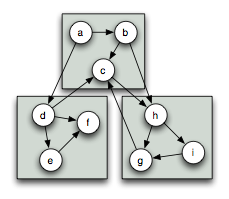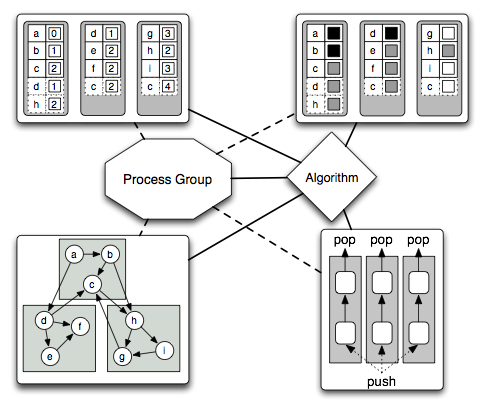#Boost C++ Libraries

...one of the most highly regarded and expertly designed C++ library projects in the world.

# An Overview of the Parallel Boost Graph LibraryThe Parallel Boost Graph Library (Parallel BGL) is a C++ library for parallel, distributed computation on graphs. The Parallel BGL contains distributed graph data structures, distributed graph algorithms, abstractions over the communication medium (such as MPI), and supporting data structures. A graph (also called a network) consists of a set of vertices and a set of relationships between vertices, called edges. The edges may be undirected, meaning that the relationship between vertices is mutual, e.g., "X is related to Y", or they can be directed, meaning that the relationship goes only one way, e.g., "X is the child of Y". The following figure illustrates a typical directed graph, where a-i are the vertices and the arrows represent edges.The Parallel BGL is primarily concerned with distributed graphs. Distributed graphs are conceptually graphs, but their storage is spread across multiple processors. The following figure demonstrates a distributed version of the graph above, where the graph has been divided among three processors (represented by the grey rectangles). Edges in the graph may be either local (with both endpoints stored on the same processor) or remote (the target of the edge is stored on a different processor).

The Parallel BGL is a generic library. At its core are generic distributed graph algorithms, which can operate on any distributed graph data structure provided that data structure meets certain requirements. For instance, the algorithm may need to enumerate the set of vertices stored on the current processor, enumerate the set of outgoing edges from a particular vertex, and determine on which processor the target of each edge resides. The graph algorithms in the Parallel BGL are also generic with respect to the properties attached to edges and vertices in a graph; for instance, the weight of each edge can be stored as part of the graph or allocated in a completely separate data structure.

The genericity available in the algorithms of the Parallel BGL allows them to be applied to existing graph data structures. However, most users will instead be writing new code that takes advantage of the Parallel BGL. The Parallel BGL provides distributed graph data structures that meet the requirements of the Parallel BGL algorithms. The primary data structure is the distributed adjacency list, which allows storage and manipulation of a (distributed) graph. The vertices in the graph are divided among the various processors, and each of the edges outgoing from a vertex are stored on the processor that "owns" (stores) that vertex. The following figure illustrates the distributed adjacency list representation.The distributed adjacency list distributes the structure of a graph over multiple processors. While graph structure is in important part of many graph problems, there are typically other properties attached to the vertices and edges, such as edge weights or the position of vertices within a grid. These properties are stored in property maps, which associate a single piece of data with each edge or vertex in a graph. Distributed property maps extend this notion to distributed computing, where properties are stored on the same processor as the vertex or edge. The following figure illustrates the distribution of a property map storing colors (white, gray, black) for each vertex. In addition to the storage for each vertex, the processors store some "ghost cells" that cache values actually stored on other processors, represented by the dashed boxes.

Tying together all of the distributed data structures of the Parallel BGL are its process groups and distributed graph algorithms. Process groups coordinate the interactions between multiple processes and distributed data structures by abstracting the communication mechanism. The algorithms are typically written using the SPMD model (Single Program, Multiple Data) and interact with both the distributed data structures and the process group itself. At various points in the algorithm's execution, all processes execute a synchronization point, which allows all of the distributed data structures to ensure an appropriate degree of consistency across processes. The following diagram illustrates the communication patterns within the the execution of a distributed algorithm in the Parallel BGL. In particular, the diagram illustrates the distributed data structures used in a distributed breadth-first search, from the top-left and proceeding clockwise:

• a user-defined property map that tracks the distance from the source vertex to all other vertices,
• an automatically-generated property map that tracks the "color" of vertices in the (distributed) graph, to determine which vertices have been seen before,
• a distributed queue, which coordinates the breadth-first search and distributes new vertices to search, and
• a distributed graph, on which the breadth-first search is operating.Copyright (C) 2005 The Trustees of Indiana University.

Authors: Douglas Gregor and Andrew Lumsdaine